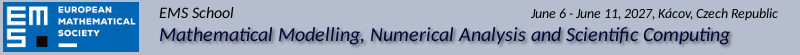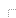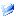Home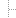ProgramParticipantsShared folderPaymentTravel InfoVenueOrganizers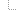History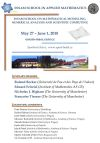flyerprogramscheme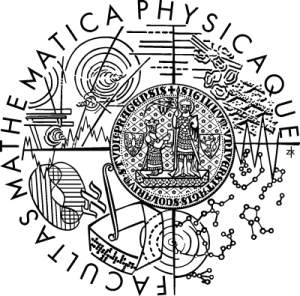Last update:
24.05.2023

# Program

The lectures will be prepared with a broad multidisciplinary audience in mind, and at each school a broad scope, ranging from modeling to scientific computing, will be covered. The four main speakers will deliver a series of three 70-minutes lectures. Ample time within the school is allocated for the promotion of informal scientific discussions among the participants.

## Speakers of the 2023 school:

The detailed program and the program scheme are available in pdf.

## Plenary speakersPatrick Farrell University of Oxford, UK Mathematical Institute Andrew Wiles Building Woodstock Road Oxford, OX2 6GG
Bifurcation techniques for calculating multiple solutions of nonlinear PDEs

Nonlinear problems often support multiple solutions, and these multiple solutions are typically very important for the application at hand. Examples include the buckling and snapping of structures, bistable devices in memory and displays, multiple local minima of nonconvex optimisation problems, multiple Nash equilibria in games, and so on. Despite their importance, the calculation of multiple solutions is not routine in many fields.

In this series of lectures I will review algorithms for calculating multiple solutions of nonlinear PDEs and exploring how they change and bifurcate as parameters in the problem are varied. Particular emphasis will be given to algorithms that scale well with problem dimension and that are simple to implement in existing Newton-based codes.Chi Wang Shu Brown University, USA Division of Applied Mathematics Box F 182 George Street Providence, RI 02912
Discontinuous Galerkin Methods: Survey and Recent Developments

In this series of lectures we will give an introduction to a class of very popular finite element methods, the so-called discontinuous Galerkin methods, for solving convection dominated partial differential equations. We will describe the algorithm formulation, present stability analysis and error estimates, and discuss practical implementation issues of the discontinuous Galerkin methods when applied to hyperbolic conservation laws, convection diffusion equations, and PDEs containing higher derivatives such as the KdV equations.

Reference: Numerical Solutions of Partial Differential Equations, S. Bertoluzza, S. Falletta, G. Russo and C.-W. Shu, Advanced Courses in Mathematics CRM Barcelona, Birkh\"{a}user, Basel, 2009 (we will only cover Part 3 on pages 149-201)Jan Zeman Czech Technical University in Prague, Czech Republic Thákurova 7 16629 Prague 6
Multi-scale computational analysis of periodic and non-periodic heterogeneous media

The series of lectures focuses on computational modeling of materials with a fine, heterogeneous microstructure. The first lecture provides an overview of homogenization results for problems with a regular microstructure characterized by a single periodic cell, using both formal asymptotic expansion as well as rigorous two-scale convergence arguments. Besides, I introduce an efficient spectral discretization technique to determine the homogenized properties of the periodic cell problem. The second lecture is dedicated to establishing a relation between the spectral approach and the method of Laplace preconditioning, which can be advantageously applied to estimating the discretization and algebraic errors when solving the cell problem. In the concluding lecture, I outline a framework for modeling of non-periodic materials based on the concept of Wang tilings and discuss a related numerical strategy that combines the ideas of generalized finite element methods, homogenization, and model order reduction.Walter Zulehner Johannes Kepler University Linz, Austria Institute of Computational Mathematics Altenberger Straße 69 A-4040 Linz
Saddle Point Problems: Construction, Analysis, and Preconditioning

Many problems from various areas of applications lead to systems of partial differential equations (PDEs) in saddle point form. This includes mixed formulations of elliptic problems as well as optimality systems for PDE-constrained optimization problems. We will discuss possible advantages of mixed formulations of elliptic problems, for example, in connection with the construction of locking-free discretization methods. We will study the flexibility of mixed formulations with respect to the choice of the involved function spaces and how exploit this flexibility for the construction of numerical methods.

It will be demonstrated that the analysis of saddle point problems in function spaces is strongly related to the construction of efficient solution procedures for the discretized problems. In particular, we will address the topic of robustness of the solution procedures with respect to certain model and numerical parameters.

The lectures will include the discussion of several examples from Computational Mechanics and examples of inverse problems resp. optimal control problems with elliptic, parabolic, or hyperbolic state equations.